## The water in a river flows uniformly at a constant speed of 2.50 m/s between parallel banks 80.0 m apart. You are to deliver a package direc

Question

The water in a river flows uniformly at a constant speed of 2.50 m/s between parallel banks 80.0 m apart. You are to deliver a package directly across the river, but you can swim only at 1.50 m/s.

(a) If you choose to minimize the time you spend in the water, in what direction should you head?

(b) How far downstream will you be carried?

(c) If you choose to minimize the distance downstream that the river carries you, in what direction should you head?

(d) How far downstream will you be carried?

in progress 0
6 months 2021-07-25T21:12:27+00:00 1 Answers 97 views 0

## Answers ( )

a)  The swimmer should travel perpendicular to the bank to minimize the spent in getting to the other side.

b) 133.33 m

c) 53.13°

d) 106.67 m

Explanation:

a) The swimmer should travel perpendicular to the bank to minimize the spent in getting to the other side.

b) velocity = distance * time

Let the velocity of the swimmer be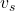= 1.5 m/s

The separation of the two sides of the river, d = 80 m

The time taken by the swimmer to get to the other end of the river bank,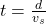t = 80/1.5

t = 53.33 s

The swimmer will be carried downstream by the river through a distance, s

Let the velocity of the river be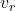= 2.5 m/s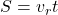S = 53.33 * 2.5

S = 133.33 m

c) To minimize the distance traveled by the swimmer, his resultant velocity must be perpendicular to the velocity of the swimmer relative to water

That is ,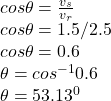d) Downstream velocity of the swimmer,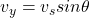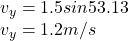The vertical displacement is given by,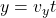80 = 1.2 t

t = 80/1.2

t = 66.67 s

the horizontal speed,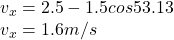The downstream horizontal distance of the swimmer,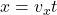x = 1.6 * 66.67

x = 106.67 m